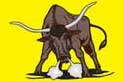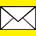### Email UsElectrical Terms
Resistor - An electrical component which offers resists the flow of electricity. The degree of this resistance is measured in ohms.
Conductivity - A measure of a materials ability to conduct an electric current.
Conductor - A material that allows electricity to flow freely. The less resistance a conductor offers, the better the conductor. Conductivity is measured in Mho (ohms spelled backwards). A mho is the reciprocal of the ohm.
Insulator - A material that will not allow any electrical current. Most insulators have a critical pressure for a given thickness. IF the critical pressure is exceeded, the insulating material will puncture.
Short - A low resistance path between live wire and dead wire or earth-return wire. Commonly known as wire-to-wire shorts. A short will compromise the reliability and effectiveness of an electric fence!
Volts - A unit of electrical pressure (very similar to how many pounds per square inch are units of physical pressure). One volt is the force required to cause a current of 1 amp to flow through a resistance of 1 ohm. Ohms x amps = volts.
Amps - A unit of electrical rate of flow (similar to how gallons per hour are units of rate of phyicsal flow). One amp is a flow rate of 6.28 x 10 to the 18th power electrons per second.
mAmps - A unit of electrical flow equal to 1/1000 of an amp (a milli amp).
Ohms - A unit of electrical resistance. One ohm is the amount of resistance that will limit the flow rate to 1 amp when a pressure of 1 volt is applied.
Coulombs - A unit of electrical quantity. One coulomb is 6.28 x 10 to the 18th power electrons, a flow rate of 1 amp for 1 second.
Watts - A unit of electrical rate of work (similar to horsepower). 46 watts equals 1 horsepower. 1 horsepower can lift 1 pound vertically at the rate of 550 feet per second. Therefore, 746 watts can lift 1 pound at the rate of 550 feet per second. A flow rate of 1 amp at a pressure of 1 volt produces 1 watt.
Joules - Units of electrical energy. One joule is the amount of energy required to produce 1 watt for 1 second.
Energy - The capacity or ability to complete a particular amount of work. It is largely the quantity of joules released by an energizer during each pulse that determines the energizers effective power.
Capacitor - An electrical component capable of storing and releasing electrical energy and approximating a reservoir, the volume of which is stated in electrical units called farads. If 1 amp flows into a capacitor for one second and this causes a rise in pressure of 1 volt, then the volume of the capacitor equals 1 farad.
Electrical Formulas
Ohms = Volts / Amps
Mhos = Amps / Volts
Volts = Ohms x Amps
Amps = Volts / Ohms
Ohms = Volts / Amps
Coulombs = Amps x Seconds
Watts = Amps x Volts
Joules = Watts x Seconds
1 kilowatt-hour = 3,600,000 Joules
Farads = Amps x Seconds/Volts Rise
1 horsepower = 46 Watts

Home | Which Charger? | AC/Plug-In Chargers | DC/Battery Chargers | Solar Chargers | About Us |